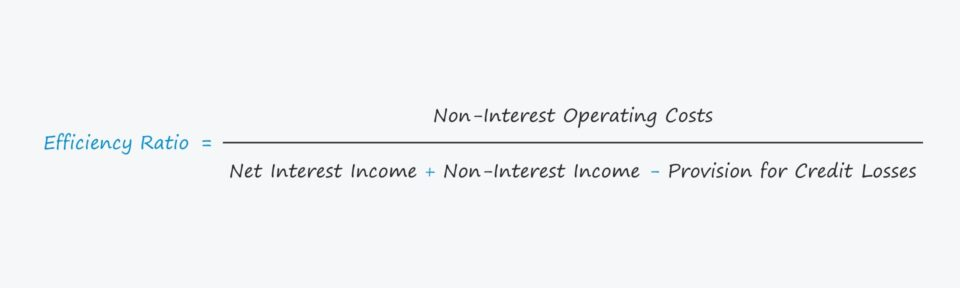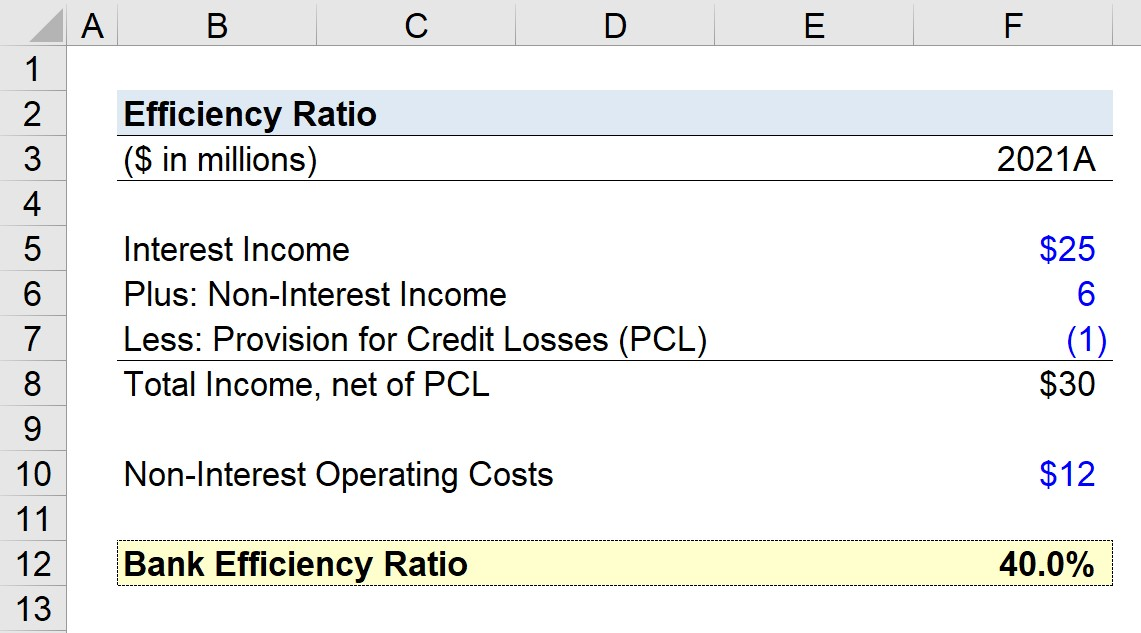Welcome to Wall Street Prep! Use code at checkout for 15% off.# Bank Efficiency Ratio

Guide to Understanding the Bank Efficiency Ratio## How to Calculate the Efficiency Ratio

The efficiency ratio is a profitability metric that can determine the operating efficiency of a bank.

Calculating the efficiency ratio involves comparing the bank’s operating expenses to its income.

The core business model of a bank is to provide loans to borrowers in exchange for interest payments and the repayment of the debt principal on the date of maturity.

The borrower, as part of the loan agreement, is contractually obligated to meet its periodic interest payments and principal repayment on time.

Thus, the revenue of a bank consists primarily of the interest payments owed by borrowers, while the costs consist of operating costs incurred to run day-to-day operations, such as:

• Employee Wages
• Office Rent
• Insurance
• Equipment and Supplies
• Infrastructure and Security

Since a bank’s financial performance is directly tied to the state of the economy (namely, prevailing market interest rates), banks must strive to reduce their operating expenses.

The operating efficiency of banks is most important during periods of economic downturns when lending volume declines and more borrowers default on their debt obligations.

## Efficiency Ratio Formula

The formula for calculating the efficiency ratio for banks is as follows.

Efficiency Ratio = Non-Interest Operating Costs ÷ (Net Interest Income + Non-Interest Income – Provision for Credit Losses)

Where:

Further details on each input can be found below.

• Non-Interest Operating Costs → The non-interest operating costs of a bank are the total expenses related to its daily business functions, excluding any costs related to interest (i.e. borrowing expenses to others).
• Net Interest Income → The net interest income is the difference between the bank’s revenue from its interest-bearing assets (e.g. loans, bonds) and the expenses related to its own interest-bearing liabilities.
• Non-Interest Income → The other source of income for banks is their non-interest income, which can come from other divisions such as sales and trading.
• Provision for Credit Losses (PCL) → The provision for credit losses, or PCL, is a deduction intended to serve as a conservative estimate of the potential losses that a company could incur from the default risk of borrowers.

## How to Interpret the Efficiency Ratio (High vs. Low)

The lower the efficiency ratio, the more efficiently the bank operates (and vice versa for higher ratios).

For the most part, larger banks tend to exhibit lower efficiency ratios because their income base is more diversified.

Because of the fact that the revenue of a larger bank is less concentrated in its lending operations, there is more of a “cushion” for it to be able to withstand recessions and underperformance.

In addition, larger banks are typically more reputable and have more optionality when it comes to picking their borrowers, i.e. such banks have a more stringent diligence process and can set higher standards for their borrowers, which directly results in less credit risk exposure (and higher recoveries in the event of default).

## Efficiency Ratio Calculator – Excel Model Template

We’ll now move to a modeling exercise, which you can access by filling out the form below.Submitting...

## Bank Efficiency Ratio Calculation Example

Suppose an institutional bank is attempting to measure its efficiency ratio for its latest fiscal year, 2021.

The total interest income the bank generated was \$25 million, along with \$6 million in non-interest income.

• Interest Income = \$25 million
• Non-Interest Income = \$6 million

Total income equals \$31 million, but we must then deduct the provision for credit losses (PCL), which was \$1 million.

• Provision for Credit Losses (PCL) = \$1 million

Upon deducting the provision for credit losses (PCL), the bank’s total income is \$30 million.

• Total Income, net of PCL = \$25 million + \$6 million – \$1 million = \$30 million

The remaining input consists of the non-interest operating costs of the bank, which we’ll assume to be \$12 million in the corresponding period.

By dividing the \$12 million in non-operating costs by the \$30 million in total income net of PCL, we arrive at an efficiency ratio of 40% for our hypothetical bank.

• Bank Efficiency Ratio = \$12 million ÷ \$30 million = 40%Step-by-Step Online Course

### Everything You Need To Master Financial Modeling

Enroll in The Premium Package: Learn Financial Statement Modeling, DCF, M&A, LBO and Comps. The same training program used at top investment banks.